##### Statistics: 1001 Practice Problems For Dummies (+ Free Online Practice)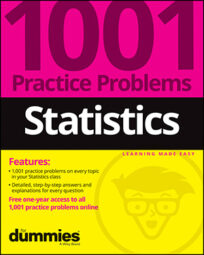You can do hypothesis tests for three specific scenarios: testing one population proportion; testing for a difference between two population proportions; and testing for a difference between two population means. Solve the following problems about testing one population proportion. Note from JM: This isn't quite accurate. You can also test one population mean.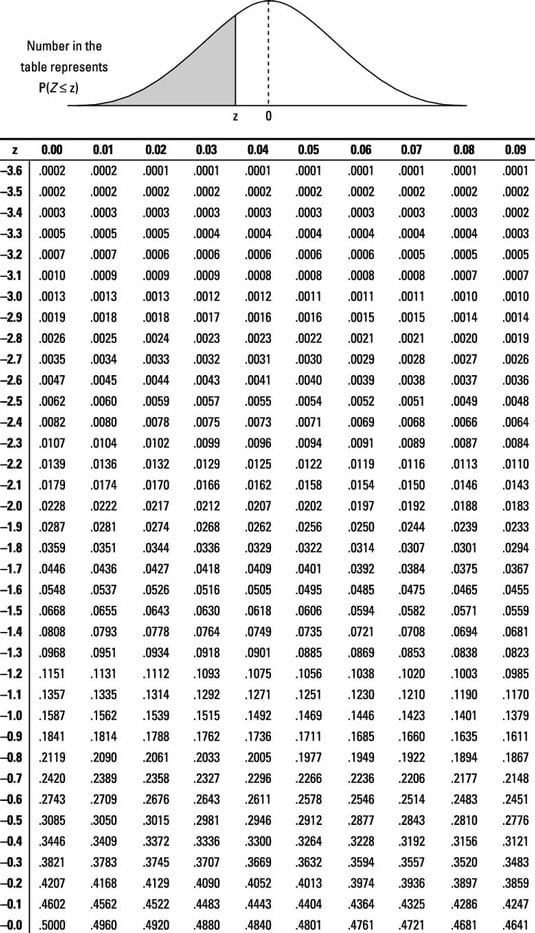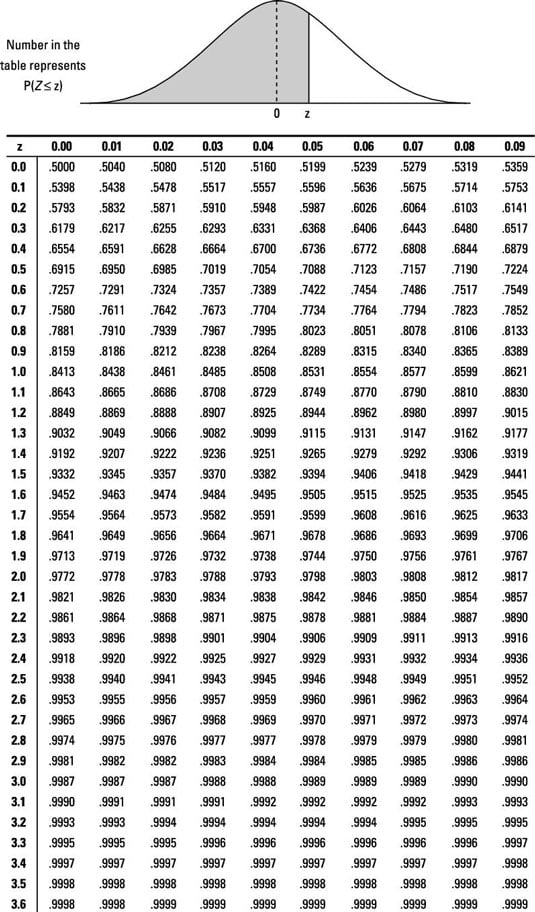## Sample questions

1. A bank will open a new branch in a particular neighborhood if it can be reasonably sure that at least 10% of the residents will consider banking at the new branch.

The bank will use a significance level of 0.05 to make its decision. The bank does a survey of residents of a particular neighborhood and finds that 19 out of 100 random people surveyed said they'd consider banking at the new branch. Run a z-test for a single proportion and determine whether the bank should open the new branch, considering its standard policy.

Answer: The null hypothesis should be rejected; the bank should open the new branch.

First, set up the null and alternative hypotheses:

H0: p0 = 0.10

Ha: p0 > 0.10

Note: Because the bank wants to ensure that at least 10% of the residents will use the new branch, it's implicitly interested in a greater than alternative hypothesis.

Next, determine whether the sample is large enough to run a z-test by checking that both np0 and n(1 – p0) equal at least 10. In this case, n = 100 and p0 = 0.10, so np0 = (100)(0.10) = 10, and n(1 – np0) = 100(1 – 0.10) = 100(0.90) = 90.

Then, compute the standard error with this formula: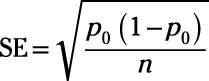where p0 is the population proportion and n is the sample size. Substitute the known values into the formula to get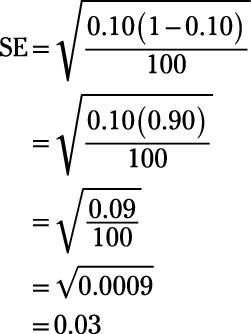Next, find the observed proportion by dividing the number who said they would consider banking at the new branch by the sample size of 100: 19/100 = 0.19.

Then, calculate the z-test statistic, using this formula: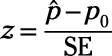where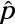is the observed proportion, p0 is the hypothesized proportion, and SE is the standard error.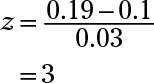Now, use a Z-table to determine the probability of observing a z-score this high or higher. Unfortunately, the table shows the probability of observing a score of z = 3.0 or lower, so you have to subtract the table probability from 1 to get the probability: 1 – 0.9987 = 0.0013.

Finally, compare the probability (that is, the p-value) with the alpha level. The bank wanted to use a significance level of 0.05, so alpha = 0.05, and the p-value of 0.0013 is much lower than that. So you reject the null hypothesis.

2. A corporate call center hopes to resolve 75% or more of customer calls through an automated computer voice recognition system. It randomly surveys 50 recent customers; 45 report that their issue was resolved.

Can the management of the corporation conclude that the computer system is hitting its minimum target, using a significance level of 0.05? Use a z-test for a single proportion to provide an answer.

Answer: The null hypothesis should be rejected; the standard has been met and exceeded.

First, set up the null and alternative hypotheses:

H0: p0 = 0.75

Ha: p0 > 0.75

Note: The alternative is a greater than hypothesis because the call center sets a minimum threshold for performance and is asking only whether the system meets or exceeds the threshold with a high degree of confidence.

Then, determine whether the sample is large enough to run a z-test by checking that both np0 and n(1 – p0) equal at least 10. In this case, n = 50 and p0 = 0.75, so np0 = 50(0.75) = 37.5, and n(1 – p0) = 50(1 – 0.75) = 50(0.25) = 12.5.

Compute the standard error with this formula:where p0 is the population proportion and n is the sample size. Substitute the known values into the formula to get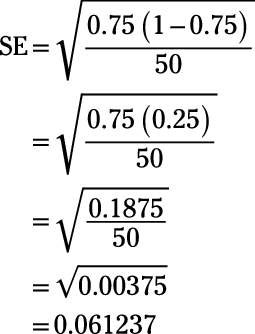Next, find the observed proportion by dividing the number of "successes" by the sample size: 45/50 = 0.90.

Then, calculate the z-test statistic, using this formula:whereis the observed proportion, p0 is the hypothesized proportion, and SE is the standard error.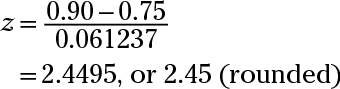Now, use a Z-table to figure out the probability of observing a value this high or higher (because the alternative is a greater than hypothesis). The table gives you the proportion of the area under the curve that's less than a given value of z, which is 0.9929. To get the desired area (proportion above this z-value), you have to subtract that number from 1: 1 – 0.9929 = 0.0071.

Finally, identify the desired alpha level (0.05) and compare the probability you found from the Z-table with that. Because 0.0071 is less than 0.05, you reject the null hypothesis.

If you need more practice on this and other topics from your statistics course, visit 1,001 Statistics Practice Problems For Dummies to purchase online access to 1,001 statistics practice problems! We can help you track your performance, see where you need to study, and create customized problem sets to master your stats skills.